Chapter 1

The electron has both an intrinsic electric field and an intrinsic magnetic field.
The electron’s intrinsic electromagnetic field has both energy and angular momentum. In our book, we have the assumption that the electron has the electromagnetic origin, the electron spin is the electron’s electromagnetic field angular momentum, and the electron’s self-energy is the electron’s electromagnetic field energy.

The simplest model of electron spin is a spinning electrically charged ball, similar to the earth rotating about its own axis. In this model, the electron’s rest energy equals the electrostatic potential energy of a sphere of charge e with radius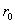: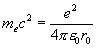?                                                                      ?/span>(1.1)

In which?/span>is the classical radius of the electron,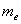?/span>is the electron’s mass.

Combine electron mass?/span>with radius, and we get electron spin angular momentum: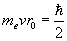(1.2)

In which v is the classical velocity for electron spin.

Then we can obtain the electron’s classical velocity: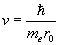(1.3)

Combine this with equation (1.1), thus: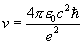(1.4)

And so: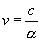?                                           ?/span>(1.5)

In which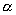?/span>is fine structure constant: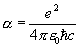? (1.6)

As we know: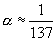? (1.7)

Therefore: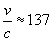?                                                        (1.8)

Based on the above model of electron spin, the classical electron velocity is much greater than the velocity of light! ?/span>Why did the spinning electric charge ball model fail for the electron spin? The basic assumption of the above electron spin model is that the electron’s mass originates from electric field energy.

In our book, we have an assumption that the electron has electromagnetic origin.?The electron’s self-energy is the electron’s electromagnetic field energy, which mainly comes from electron magnetic field energy, in comparison to the electron’s magnetic field energy, the electron’s electric field energy is much smaller. This is one of the major reasons why the above electron spin model failed.

We no longer regard the electron as a point-like particle. Instead, we assume that electrons have internal structure; inside the electron it has continuum spherical distribution of both electric charge and magnetic charge. Based upon the charge distribution, and also according to the Gauss Laws for electric field and magnetic field, we calculate the electric field and magnetic field distribution inside the electron, and then we calculate electromagnetic field energy and angular momentum of the electron.

Then we make the assumption that both the electron mass and spin have electromagnetic origin. Thus we obtain the electron’s self-energy from the electromagnetic field energy, and the electron spin from the electron’s electromagnetic field angular momentum.

Then we extend our electron’s electromagnetic model to the proton and neutron, the proton and neutron also have electromagnetic origin; and we continue our electromagnetic model to the hydrogen and helium atoms in our book.

In our book, we also provide a possible solution for the hydrogen atom spectrum, in which the hydrogen atom spectrum is regarded as the hydrogen electromagnetic field stationary wave energy spectrum.

The particles that comprise all physical materials include electrons, protons and neutrons; all of which have electromagnetic origin. Therefore, all materials have electromagnetic origin.

 What is the electron spin? ISBN 0-9743974-9-0 Copyright ?2003 Gengyun Li All rights reserved http://www.electronspin.org